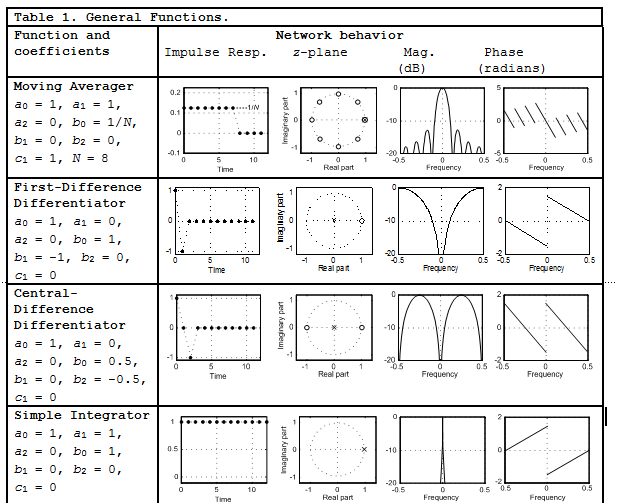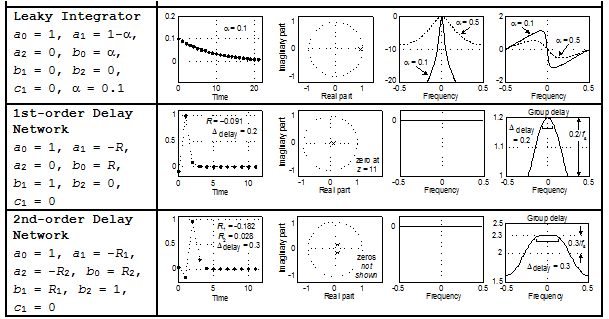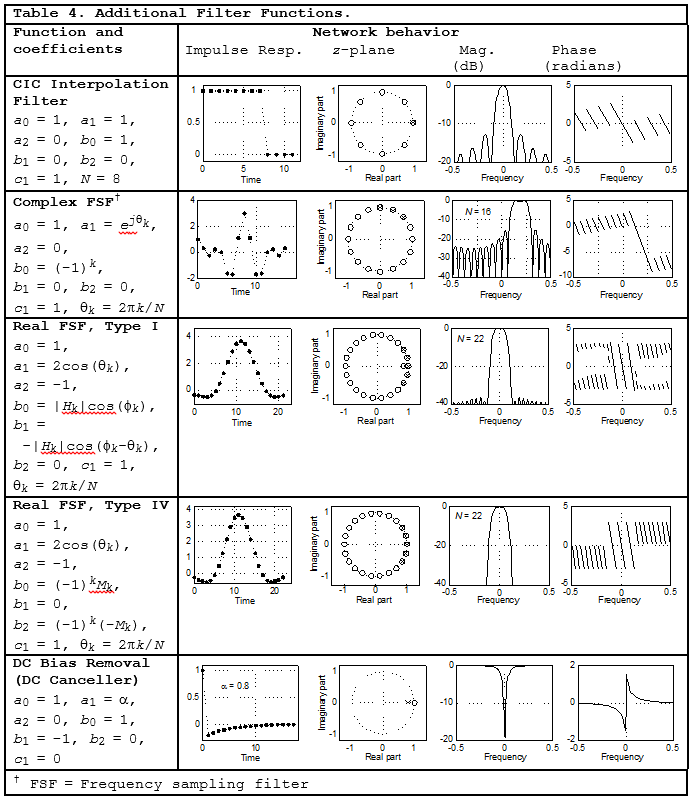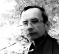# The Swiss Army Knife of Digital Networks

This blog describes a general discrete-signal network that appears, in various forms, inside so many DSP applications.Figure 1 shows how the network's structure has the distinct look of a digital filter—a comb filter followed by a 2nd-order recursive network. However, I do not call this useful network a filter because its capabilities extend far beyond simple filtering. Through a series of examples I've illustrated the fundamental strength of this Swiss Army Knife of digital networks and its ability to be reconfigured to perform an astounding number of useful functions based on the values of its seven control coefficients.Figure 1. General discrete-signal processing network.

The Figure 1 network has a transfer function of

$$H(z) = \frac{Y(z)}{X(z)} = (1-c_1z^{-N})\frac{b_0 + b_1z^{-1} + b_2z^{-2}}{1/a_0 - a_1z^{-1} - a_2z^{-2}} \tag{1}$$

The tables in this blog list various signal processing functions performed by the network based on the $a_n,\ b_n\ and\ c_1$ coefficients. Variable $N$ is the order of the comb filter. Included in the tables are depictions of the network's impulse response, z-plane pole/zero locations, as well as frequency-domain magnitude and phase responses. The frequency axes in those tables is normalized such that a value of 0.5 represents a frequency of $f_s / 2$ where $f_s$ is the sample rate in Hz.

To keep this blog manageable in size, detailed explanations of the network Functions in the following tables are contained in the downloadable PDF file associated with this blog.

[NOTE FROM AUTHOR LYONS (Oct. 2018): The downloadable PDF file has been updated since this blog’s original posting in June 2016.]Previous post by Rick Lyons:
Digital Envelope Detection: The Good, the Bad, and the Ugly
Next post by Rick Lyons:
An Astounding Digital Filter Design Application

[ - ]
Comment by June 13, 2016Rick:

I don't see any provisions for integrator anti-windup.

:)
[ - ]
Comment by June 15, 2016Making the integrator slightly leaky would be fine for the moving averager and other a_1 = 1 configurations. Shouldn't be much of a difference, just a slight decay in moving average window. At least the comb is before the integrator!
[ - ]
Comment by July 3, 2016Pole-zero diagrams are useful.
May be better to explain the equaliser as an allpass filter.

To post reply to a comment, click on the 'reply' button attached to each comment. To post a new comment (not a reply to a comment) check out the 'Write a Comment' tab at the top of the comments.